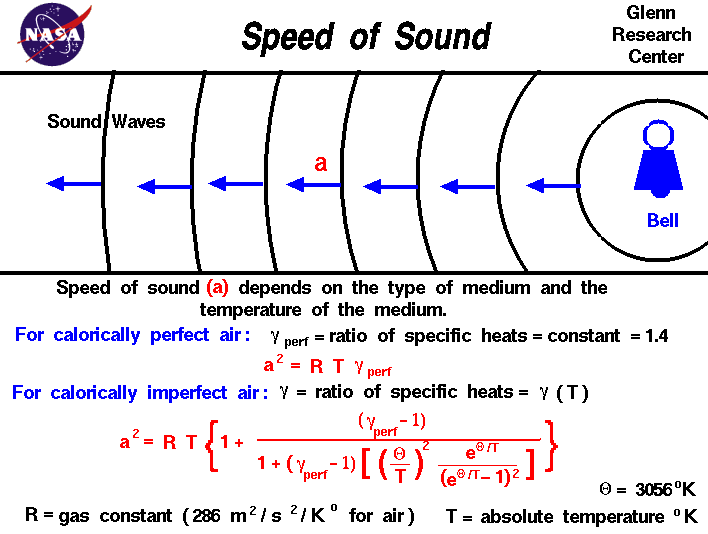# Speed Of Sound Study Guide

INTRODUCTIONSource

Sound speed is the rate at which sound waves travel through various materials. The contemporary value for the speed of sound in dry air at a temperature of 0 °C (32 °F) is 331.29 meters (1,086.9 feet) per second.

## SOUND SPEED IN DIFFERENT MEDIUMS

• Sound’s speed in colloquial speech refers to the speed of sound waves in the air.
• Sound travels at different speeds in different substances.
• Gases are the slowest, liquids are faster, and solids are the fastest.
• While sound travels at 343 meters per second in air, it travels at 1,481 meters per second in water (almost 4.3 times faster) and 5,120 meters per second in iron (almost 15 times as fast).
• Sound travels at approximately 12,000 m/s through a very hard solid like a diamond, nearly 35 times its speed in air and the fastest it can travel under normal conditions.

## FACTORS AFFECTING THE SPEED OF SOUND

The speed of sound is affected by the density and temperature of the material through which it travels.Source

### THE MEDIUM’S DENSITY

When the medium is dense, the molecules are packed tightly together, allowing sound to travel faster. As a result, as the density of the medium increases, so does the speed of sound.

### THE MEDIUM’S TEMPERATURE

The temperature has a direct relationship with the speed of sound. As a result, the speed of sound increases as the temperature rises.

## SPEED OF SOUND FORMULA

Because the speed of sound is defined as the distance traveled by a sound wave in a given amount of time, it can be calculated using the following formula:

v = λ f

Where v denotes velocity,  λ  denotes sound wave wavelength, and f denotes frequency.

For all waves, the relationship between the speed of sound, its frequency, and wavelength is the same. The distance between neighboring compressions or rarefactions determines the wavelength of a sound. The quantity of waves that pass a location per unit of time has the same frequency as the source.

Example

How long does a sound wave with a frequency of 2 kHz and a wavelength of 35 cm take to traverse 1.5 kilometers?

Solution:

We know that the formula for calculating sound speed is:

v = λ v

We get by substituting the numbers in the equation.

v = 0.35 m × 2000 Hz = 700 m/s

The time it takes a sound wave to traverse 1.5 kilometers can be calculated as follows:

Time = Velocity/Distance Traveled

We get this by substituting the numbers in the equation.

Time = 1500 meters / 700 meters per second = 2.1 seconds

# SUMMARY

• The speed of “sound” is actually the rate at which a minor disturbance is transmitted across a medium.
• The contemporary value for the speed of sound in dry air at a temperature of 0 °C (32 °F) is 331.29 meters (1,086.9 feet) per second.
• Sound travels at different speeds in different substances: sound travels the slowest in gases, the fastest in liquids, and the fastest in solids.

## FAQs

Q. What can affect the speed of sound?

The speed of sound is affected by the density and temperature of the material through which it travels.

Q. What limits the speed of sound?

Because sound is a wave that propagates by causing surrounding particles to interact, its speed is determined by the density of a material and the way its atoms are bonded together. Atoms can only travel so fast, and that movement limits the speed of sound.

Q. Does temperature affect the speed of sound?

Yes, the temperature of the material affects the sound’s speed.

Q. Does pressure affect the speed of sound?

The speed of sound is unaffected by gas pressure at a constant temperature.

We hope you enjoyed studying this lesson and learned something cool about Speed of Sound! Join our Discord community to get any questions you may have answered and to engage with other students just like you! We promise, it makes studying much more fun!😎

## REFERENCE

1. Speed of Sound: https://byjus.com/physics/speed-of-sound-propagation/ Accessed 14th April 2022.
2. Speed of Sound: https://en.wikipedia.org/wiki/Speed_of_sound  Accessed 14th April 2022.
]]>